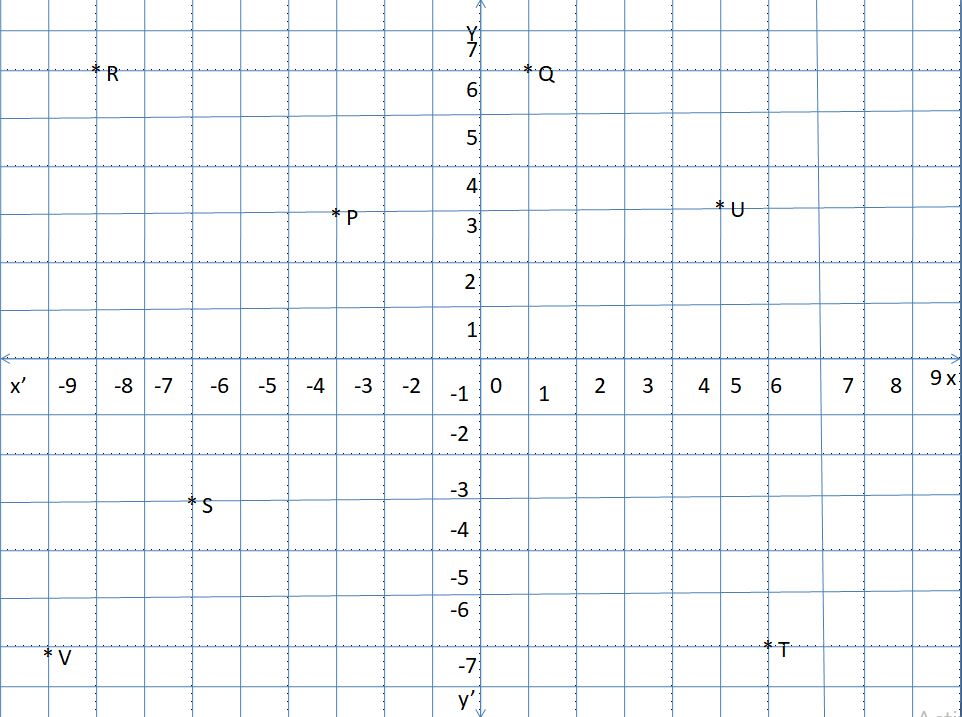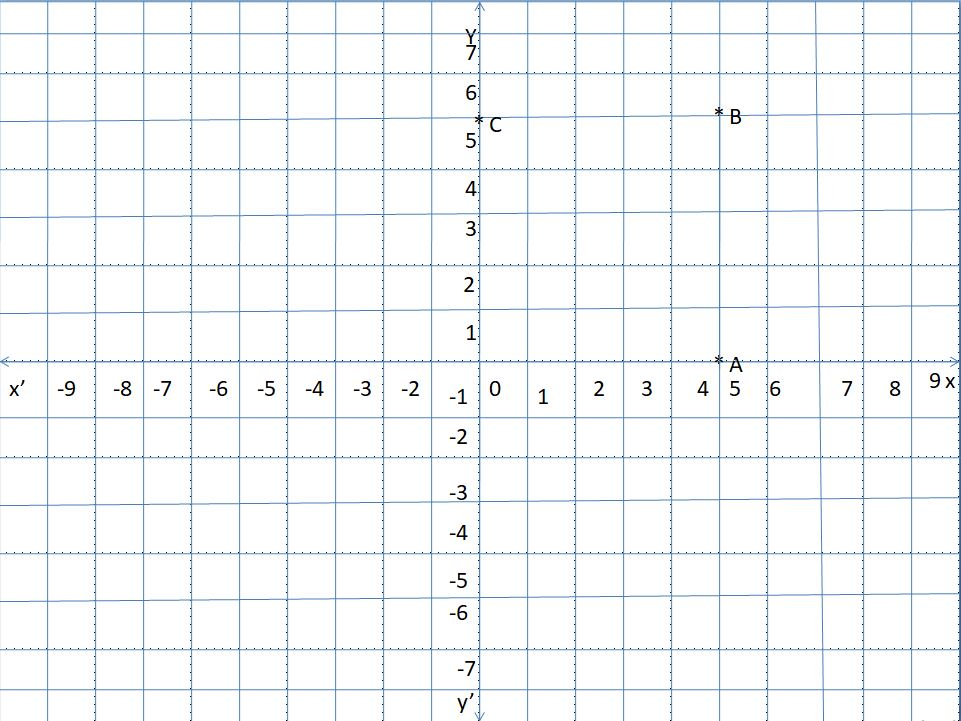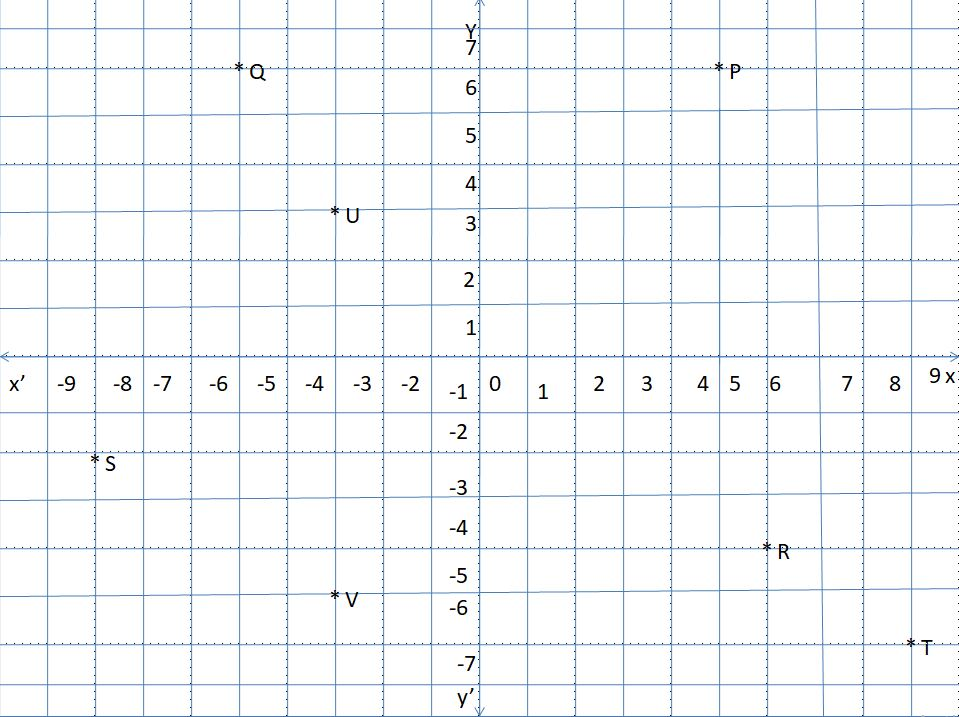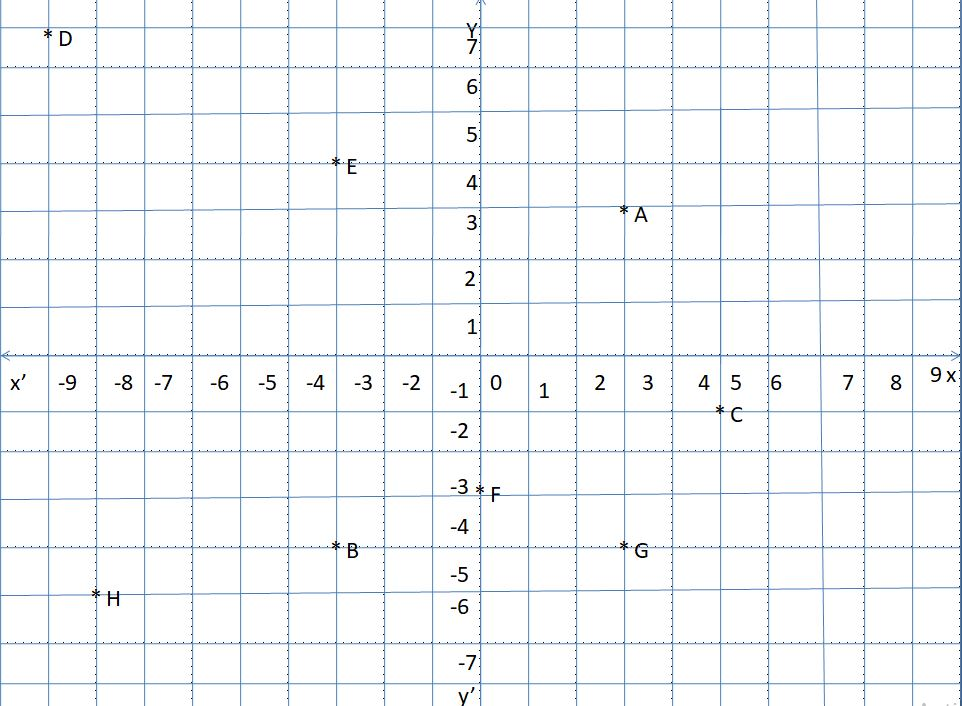# Worksheet on Coordinate Graph | Questions Based on Coordinate Graph

Worksheet on Coordinate Graph is the best source to begin your math practice. All questions related to the cartesian plane are given here to help the students learn maths. Simply check the solved questions on all four quadrants, signs of coordinates, plot points, graphs of simple function, etc. Get to know how to solve the coordinate graph questions simply by referring to this page.

For the sake of your comfort, we even listed the step by step solutions to all the sample problems provided. Solve the coordinate graph questions on your own and verify with the answers provided and test your preparation level.

1. What is the x coordinate, y coordinate of the following ordered pairs?

(i) (0, 8)

(ii) (5, -7)

(iii) (-8, 2)

(iv) (6, 7)

(v) (-9, -15)

Solution:

(i) (0, 8)

Here, the x coordinate is 0, y coordinate is 8. The point lies on the y-axis.

(ii) (5, -7)

Here, the abscissa is 5, the ordinate is -7. So, the point lies in the fourth quadrant.

(iii) (-8, 2)

Its x coordinate is -8, y coordinate is 2. So, the point lies in the second quadrant.

(iv) (6, 7)

Its x coordinate is 6, y coordinate is 7. The point lies in the first quadrant.

(v) (-9, -15)

Its x coordinate is -9, y coordinate is -15. So, this ordered pair lies in the third quadrant.

2. In which quadrant do the following points lie?

(i) (-16, 25)

(ii) (58, -36)

(iii) (27, 42)

(iv) (-36, 49)

(v) (-81, -150)

Solution:

(i) (-16, 25)

As this point abscissa is negative, and the ordinate is positive, the point lies in quadrant II.

(ii) (58, -36)

The x coordinate is positive, y coordinate value is negative. So, the point lies in the fourth quadrant.

(iii) (27, 42)

Both the coordinates of the ordered pair are positive. So, the point lies in quadrant I.

(iv) (-36, 49)

This point x coordinate value is negative, y coordinate value is positive. So, the point lies in the second quadrant.

(v) (-81, -150)

As this point both coordinates are negative values, the point lies in the third quadrant.

3. Find which of the following points lies on the x-axis. And give a reason.

(i) (0, 8)

(ii) (29, 0)

(iii) (0, 0)

(iv) (-45, 0)

(v) (0, 26)

(vi) (52, -96)

(vii) (82, 0)

(viii) (-10, 0)

Solution:

If the point has a y coordinate value is 0, then that point lies on the x-axis.
The list of points which lies on the x-axis are (ii) (29, 0), (iv) (-45, 0), (vii) (82, 0), and (viii) (-10, 0).

4. Find which of the following points lies on the y-axis. And give a reason.

(i) (0, 52)

(ii) (0, 75)

(iii) (-96, 95)

(iv) (88, 0)

(v) (0, -26)

(vi) (36, 72)

(vii) (96, 0)

(viii) (-63, 0)

Solution:

If the point has an x coordinate or the abscissa value is 0, then that point lies on the y-axis.
The list of points that lies on the y-axis are (i) (0, 52), (ii) (0, 75), (v) (0, -26).

5. Plot the following points on a graph paper.

(i) (5, 6)

(ii) (-8, -6)

(iii) (2, -7)

(iv) (0, 3)

(v) (-1, 5)

(vi) (6, 0)

(vii) (5, 4)

Solution:

Draw X’OX, Y’OY on a graph paper.

(i) The point (5, 6) lies in the first quadrant. And take 5 units along the x-axis towards the right from the origin, 6 units along the y-axis upwards from the origin to get the point.

(ii) As this point both coordinates are negative, it lies in the third quadrant. Take 8 units along the x-axis towards the right from the origin, 6 units along the y-axis downwards to plot the point (-8, -6).

(iii) This point lies in the fourth quadrant. Take 2 units on the x-axis, -7 units on the y-axis to get the point (2, -7).

(iv) This ordered pair x coordinate is zero. So the point lies on the y-axis. Take 3 units on the y-axis to get the point (0, 3).

(v) Point lies in the second quadrant. Take -1 unit along the x-axis, 5 units along the y-axis to get the point (-1, 5).

(vi) This point y-coordinate is 0. So, the point lies on the x-axis. Take 6 units along the x-axis and mark that point as (6, 0).

(vii) Both coordinates of the point lies in the first quadrant. Take 5 units along the x-axis, 4 units along the y-axis to plot the point (5, 4).6. Tick (✔) the correct answer in each of the following:

(i) Which of the following quadrants does point A (56, -82) lie?

(ii) Which of the following quadrants does point B (56, 82) lie?

(iii) Which of the following quadrants does point C (-56, -82) lie?

(iv) Which of the following quadrants does point D (-56, 82) lie?

(v) The abscissa of a point is its distance from the

(a) origin

(b) x-axis

(c) y-axis

(d) none of these

(vi) The ordinate of a point is its distance from the

(a) origin

(b) x-axis

(c) y-axis

(d) none of these

(vii) Which of the following equation represents the x-axis?

(a) x = a

(b) y = 0

(c) x = y

(d) x = 0

(viii) What is the other name for abscissa?

(a) y coordinate

(b) ordinate

(c) x coordinate

(d) none of these

Solution:

(i) Point A (56, -82) lies in Quadrant IV i.e d. Because x coordinate value is positive, y coordinate is negative.

(ii) Point B (56, 82) lies in quadrant I i.e a. Because both coordinates are positive.

(iii) Point C (-56, -82) lies in quadrant III i.e c. Because both coordinates are negative.

(iv) Point D (-56, 82) lies in quadrant II i.e b. Because x coordinate is negative, y coordinate value is positive.

(v) The abscissa of a point is its distance from the origin.

(vi) The ordinate of a point is its distance from the origin.

(vii) The equation x = 0 represents the x-axis.

(viii) Another name for abscissa is x coordinate.

7. XOX’ and YOY’ are the co-ordinate axes. Find out the co-ordinate of points, P, Q, R, S, T, U, and V. Also write x coordinate and y coordinate for each point.Solution:

From the coordinate graph, we can find the coordinates of the points P, Q, R, S, T, U, and V.

P coordinates are (-3, 3). Its x coordinate value is -3, y coordinate value is 3.

Q coordinates are (1, 6). Its abscissa value is 1, the ordinate value is 6.

R coordinates are (6, -8). Its x coordinate value is 6, y coordinate value is -8.

S coordinates are (-6, -3). Its x coordinate is -6, y coordinate is -3.

T coordinates are (6, -6). Its abscissa is 6, ordinate is -6.

U coordinates are (5, 3). Its x coordinate is 5, y coordinate is 3.

V coordinates are (-9, -6). Its x coordinate is -9, y coordinate is -6.

8. Plot the points P (4, 0), Q (8, 3), R (2, 6), S (-7, 5), T (3, -1) on the graph.

Solution:

The point P (4, 0) lies on the x-axis. Point Q (8, 3) lies in the first quadrant, point R (2, 6) lies in the first quadrant. The point S (-7, 5) lies in quadrant II, T (3, -1) lies in quadrant IV.
Draw two perpendicular lines represents the x-axis, y-axis. Plot these points on the graph.9. On which axis do the following points lie.

(i) (0, 53)

(ii) (0, 0)

(iii) (86, 0)

(iv) (42, 63)

(v) (0, 52)

(vi) (71, 0)

(vii) (289, 0)

Solution:

Any points whose x coordinate value is 0, then that point lies on the y-axis. If a point y coordinate value is 0, then that point lies on the x-axis.

So, from the above list, (0, 53), (0, 52) lies on the y-axis. The points (86, 0), (71, 0), (289, 0) lies on the x-axis.

The point (0, 0) is called the origin which is the midpoint of both axes. In the point (42, 63) none of the coordinate values is 0, hence it does not lie on the axes.

10. Plot points A (5, 0), B (5, 5), C (0, 5). And join OA, AB, BC, OC. What figure do you get?

Solution:

Given points are A (5, 0), B (5, 5), C (0, 5).

Plot those points on the graph having X’OX, Y’OY as axes. Join those points.By joining the points, we will get a square having 5 units side length.

11. Write the co-ordinates of the points P, Q, R, S, T, U, V marked on the graph paper.Solution:

The coordinates of P are (5, 6). Its x coordinate is 5, y coordinate is 6.

Q coordinates are (-5, 6). Its abscissa is -5, ordinate is 6.

R coordinate values are (6, -4). Its x coordinate is 6, y coordinate is -4.

S coordinates are (-2, -8). Its abscissa is -2, ordinate is -8.

T coordinate values are (9, -6). Its x coordinate is 9, y coordinate is -6.

U coordinate values are (-3, 3). Its x coordinate is -3, y coordinate is 3

V coordinate values are (-3, -5). Its x coordinate is -3, y coordinate is -5.

12. Write the following by observing the adjoining figure.(i) Co-ordinates of G

(ii) Point identified by (-1, 5)

(iii) Coordinates of B

(iv) Point identified by (3, 3)

(v) Abscissa of point H

(vi) Ordinate of point E

(vii) Abscissa of point E

(viii) Co-ordinates of point F

(ix) Point identifies by (-9, 7)

(x) Point having the coordinates (-3, -4)

Solution:

(i) The coordinates of G are (3, -4).

(ii) Point identified by coordinates (-1, 5) is C.

(iii) The coordinates of B are (-3, -4).

(iv) The point identified by (3, 3) is A.

(v) The abscissa of point H is -8.

(vi) The ordinate of point E is -3.

(vii) The abscissa of point E is 4.

(viii) The coordinates of point F are (0, -4)

(ix) The point identified by (-9, 7) is D.

(x) The point having coordinates (-3, -4) is B.

13. (a) Draw the graph of the function y = 2x.

(b) From the graph, find the value of y, when (i) x = 1.5, (ii) x = -2, (iii) x = 2.5.

Solution:

Given function is y = 2x

Find the value of y for the corresponding values of the x.

If x = 0, then y = 2 * 0 = 0

If x = 1, then y = 2 * 1 = 2

If x = -1, then y = 2 * -1 = -2

If x = 2, then y = 2 * 2 = 4

x 0 1 -1 2
y = 2x 0 2 -2 4

Plot the points O (0, 0), A (1, 2), B (-1, -2), C (2, 4) on the graph paper.

Join those points to get a line equation.Linear equation forms a straight line.

By observing the graph, the value of y when x = 1.5 is 3, -2 is -4, 2.5 is 5.

14. Draw the graph of the function A = x4. From the graph, find the value of A, when (i) x = 2.5, (ii) x = 0, (iii) x = 3.5.

Solution:

Given function is A = x4

Substitute x =1, 2, 3 in the function to get the A value.

If x = 1, then A = 14 = 1

If x = 2, then A = 24 = 16

If x = 3, then A = 34 = 81

x 1 2 3
A 1 16 81

Now plot the points A (1, 1), Q (2, 16), C (3, 81) on a graph paper.

Join those points to get a line equation.By observing the graph, the value of y when x = 2.5 is 39, 0 is 0, 3.5 is 150.

15. Draw a graph of distance vs time. When the distance covered by an object is 3 times the time taken. Find the distance covered at 3 minutes, 4 minutes?

Solution:

Given that,

When T = 0.5, D = 3 * 0.50 = 1.5

When T = 1, D = 3 * 1 = 3

When T = 1.5, D = 3 * 1.5 = 4.5

When T = 2, D = 3 * 2 = 6

When T = 2.5, D = 3 * 2.5 = 7.5

x 0.5 1 1.5 2 2.5
A 1.5 3 4.5 6 7.5

Now plot the points A (0.5, 1.5), B (1, 3), C (1.5, 4.5), D (2, 6), E (2.5, 7.5) on the graph.
Join those points to get a graph equation.

Take time along the x-axis, distance along the y-axis.

On the x-axis: 1 unit = 30 seconds.

On the y-axis: 1 unit = 1 unit.Reading off the values from the graph.

On the x-axis, take the point L at t = 3.

Draw LP ⊥ x-axis, meeting the graph at P.

Clearly, PL = 9 units.

Therefore, t = 3 ⇒ D = 9.

On the x-axis, take the point M at t = 4

Draw MQ ⊥ y-axis, meeting the graph at Q.

Clearly, QM = 12 units.

Therefore, t = 4 ⇒ D = 12.